# NCERT Solutions for Class 8 Maths Chapter 1 Rational Numbers

NCERT Solutions for Class 8 Maths Chapter 1 Rational Numbers

### NCERT Solutions for Class 8 Maths Chapter 1 Rational Numbers

NCERT Solutions for Class 8 Maths Chapter 1 Rational Numbers Exercise 1.1

Ex 1.1 Class 8 Maths Question 1.
Using appropriate properties find: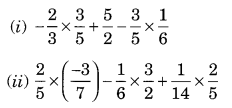Solution:Ex 1.1 Class 8 Maths Question 2.
Write the additive inverse of each of the following:Solution: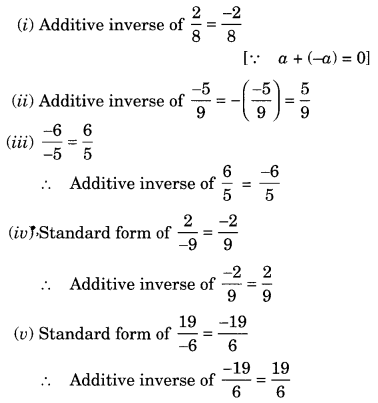Ex 1.1 Class 8 Maths Question 3.
Verify that -(-x) = x forSolution: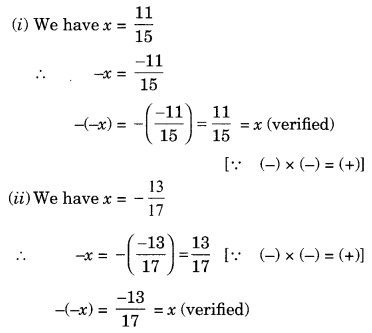Ex 1.1 Class 8 Maths Question 4.
Find the multiplicative inverse of the following:Solution: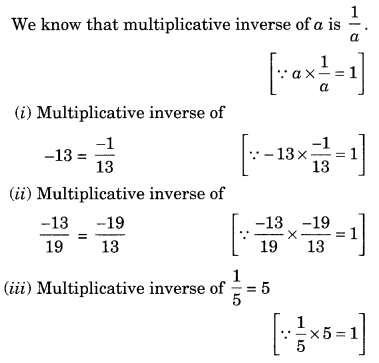Ex 1.1 Class 8 Maths Question 5.
Name the property under multiplication used in each of the following: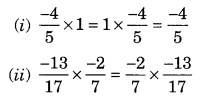Solution:
(i) Commutative property of multiplication
(ii) Commutative property of multiplication
(iii) Multiplicative inverse property

Ex 1.1 Class 8 Maths Question 6.Solution: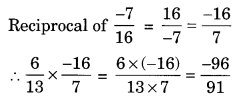Ex 1.1 Class 8 Maths Question 7.
Tell what property allows you to computeSolution:
Since a × (b × c) = (a × b) × c shows the associative property of multiplications.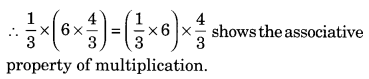Ex 1.1 Class 8 Maths Question 8.Ex 1.1 Class 8 Maths Question 9.Solution:Ex 1.1 Class 8 Maths Question 10.
Write:
(i) The rational number that does not have a reciprocal.
(ii) The rational numbers that are equal to their reciprocals.

(iii) The rational number that is equal to its negative.
Solution:
(i) 0 is the rational number which does not have its reciprocalEx 1.1 Class 8 Maths Question 11.
Fill in the blanks.
(i) Zero has ……….. reciprocal.
(ii) The numbers ……….. and ……….. are their own reciprocals.
(iii) The reciprocal of -5 is ………
(iv) Reciprocal of 1x, where x ≠ 0 is ……….
(v) The product of two rational numbers is always a …………
(vi) The reciprocal of a positive rational number is ……….
Solution:
(i) no
(ii) -1 and 1
(iii) 1/5
(iv) x
(v) rational number
(vi) positive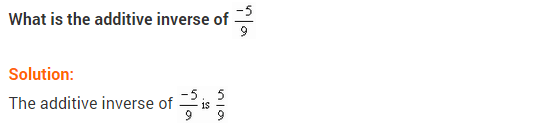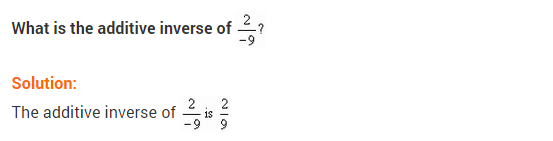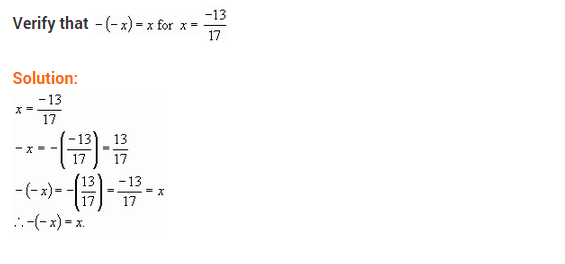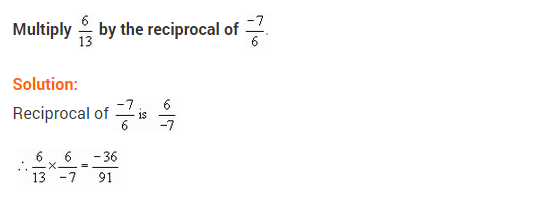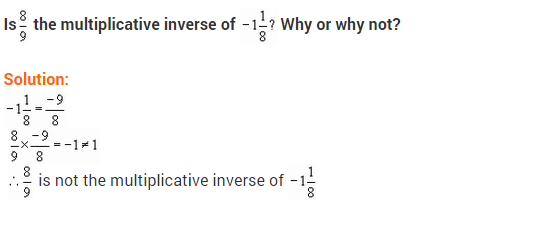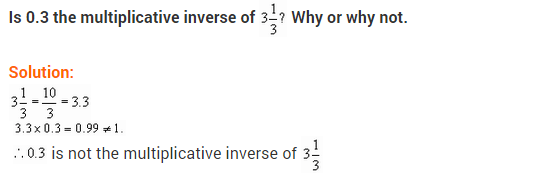## SabDekho

The Complete Educational Website